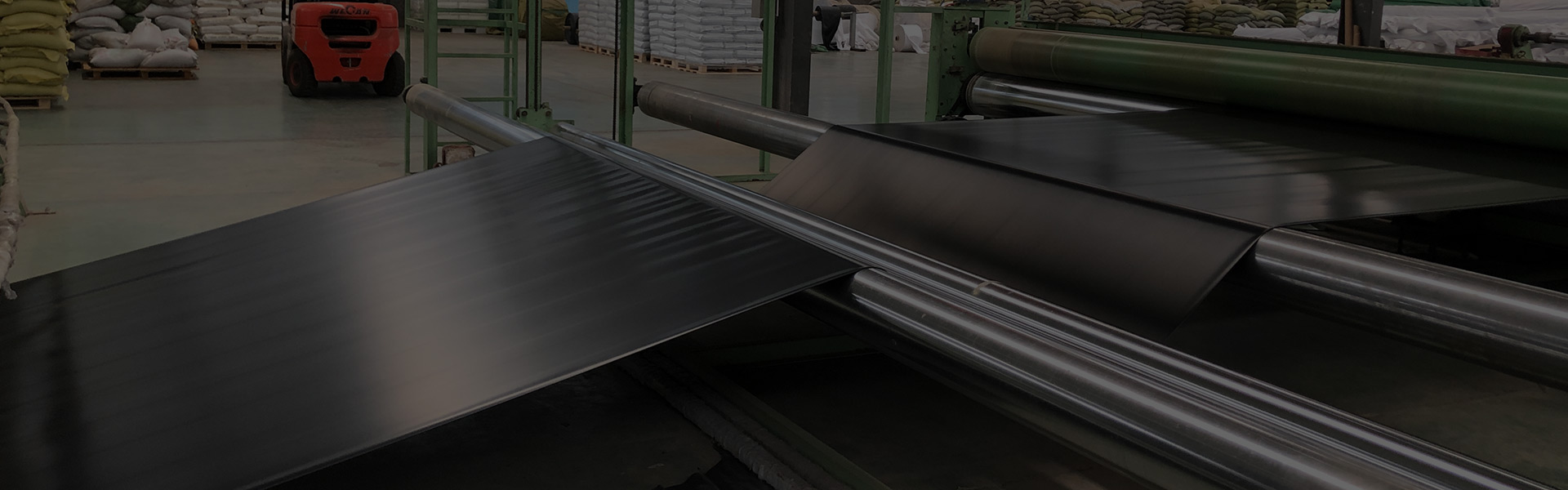zixun

# 高速公路悬臂连续梁挂篮设计与施工

1、工程概况： 跨A7高速公路连续梁。梁体为单箱室、变高度、变截面结构。箱梁顶宽12.0m，箱梁底宽6.7m，。顶板厚度除梁端附近外均为40cm，底板厚度40至100cm，按直线线性变化，腹板厚48至60、60至90cm，按折线变化。本桥梁段长度分为三种，分别为2.7m、3.1m、3.5m。主桥连续梁采用悬臂浇注挂篮施工方法，其中最重的梁段为1#梁块重136.882t。

2、挂篮型式的选择 挂篮形式多种多样，目前主要的有：三角挂篮、平行挂篮、贝雷挂篮等。我们在查找大量挂篮资料和咨询有类似施工经验的人员，同时考虑到本桥的结构特点、设计图纸中的有关要求、自身的类似施工经验以及经济适用等，我部最终决定采用以槽钢为主桁梁的三角挂篮悬浇挂篮。

3、挂篮的设计思路及荷载选择 此套挂篮按照取最重梁块的重量，考虑到不同长度梁块的施工的情况，再考虑现场所能使用的各种材料进行设计，计算荷载始终取最大和最不利的进行验算。该套挂篮的结构偏重于安全。 每个挂篮承受荷载主要有三种：梁段的自重G1 为136.882t、单套挂篮和模板的重量G2为57.2t、施工荷载G3，总重G＝G1＋ G2＋G3，G1取挂篮施工中最重一块梁段自重， G3施工荷载，取值随工艺而变，根据用泵送混凝土实测0.1t/㎡（取梁顶面面积）取值。

4、结构计算

（一）、承重体系 承重系统包括主构桁架、上横梁、下横梁、下纵梁。每只挂篮有2组主构桁架,每组主构桁架为2组双拼I25b工字型钢主纵梁,2组双拼［30型钢立杆、2根双拼［30型钢前拉杆,2根双拼［30型钢后拉杆,组成三角形稳定体系,是主要的受力结构。每只挂篮的主构桁架共设上主纵梁2组。∠10*10角钢栓接组合成竖向及斜向门架在立杆顶部连接及后拉杆以加强其横向稳定性。前上横梁采用双拼I45b型钢拼装组成,长8米,栓接于主梁上翼缘;前后下横梁均采用单拼I45b型钢,型钢梁的中间均采用10毫米厚的钢板加筋肋,前、后下横梁长度均为8米。

（二）、锚固系统 锚固系统位于组合导梁的后部,利用已浇梁体的竖向预应力钢筋和已浇梁自重来锚固。用已浇梁体上竖向预应力筋,并使用预应力筋生产商配套接头和接长筋及锚固系统进行锚固,使挂篮组合导梁与已浇梁体连接固定,通过预应力筋固定挂篮根部主纵梁。预应力筋为冷拉25毫米精轧螺纹钢筋,Fpk=830MPa,设计张拉强度为0.812Ryb=638MPa,张拉控制力为F=513KN。且等挂篮前移后再张拉至设计要求的应力值。丝口搭接大于5厘米,锚固丝口长度高于锚固端3丝以上,并通过固定导梁,保证挂篮在施工荷载下,不致发生倾覆,以确保挂篮的安全施工。 　　本工程每个导梁下设3根后锚螺杆(预应力精轧螺纹筋和连接器连接),一只挂篮为9根后锚螺杆,端部垫10×10×1.5厘米垫板,后备高强度的配套螺母锚件。

(三)、行走系统 挂篮行走系统采用在已浇筑梁体内预埋φ16圆钢弯成U行并末端弯成标准弯钩。在导梁上面放置四根φ16圆钢以便利于行走，在导梁每10cm画一个刻度线，便于观察两个挂篮行走距离是否相等，如发现不相等应及时调整。在挂篮行走过程中,为保证挂篮就位的顺利进行,防治因挂篮前端自重产生不平衡弯矩而发生倾覆,在立杆顶处设置2根10吨葫芦组成缆风,后拉在箱梁体预埋件上,进一步确保挂篮在行走时不发生倾覆。

（四）、模板系统 模板系统包括底模系统、外模系统、内模系统。模板系统要注重混凝土外观质量和底模外线型的可调性,以刚度控制设计。 　　底模系统在混凝土悬臂施工中承受钢筋混凝土重量及施工机具重量,并兼作施工操作平台。由下纵梁及底模板组成。底模板采用5毫米厚钢板加筋,具有光洁度好、平整度好、强度高、板厚均匀、相邻两板高度差小等优点。用2米靠尺量时,底板平整度偏差不得大于2毫米。　　外侧模采用定制钢模板,形成一个三角形模板体系,长度4米,可往外延伸,高度6.678米(1#块梁高)可往下延伸,同时吊挂于已浇捣的翼板上,梁腹板内外模用螺栓连接。挂篮移动前,解除箱梁翼板内侧精轧螺纹钢,使外行走梁往下移动一定距离后往外和往下延伸模板,模板内侧搁置在外行走梁上,挂篮移到位后,再定位模板,安装翼板内侧精轧螺纹钢。

（五）、挂蓝结构验算1、底模纵向分配梁受力验算 底模纵向分配梁采用型钢I36b,在腹板范围按间距30cm布置,底板范围按间距60cm布置。经计算,在浇注首个3.5m节段(4#块)时其受力最为不利。　a、腹板下纵向分配梁受力情况: 　　混凝土荷载(前端):qf1=4.918×2.6×0.4=5.11t/m 　　混凝土荷载(后端):qf1=4.592×2.6×0.4=4.77t/m 　　模板荷载:qf2=0.2×0.4=0.08t/m 　　施工及人行荷载:qL1=(0.2+0.2)×0.4=0.16t/mMmax=3.965t.m,Qmax=4.532t 　　b、底板下纵向分配梁受力情况 　　混凝土荷载(前端):qd1=5.9×2.6×1=15.34t/m 　　混凝土荷载(后端):qd1=5.9×2.6×1=15.34t/m 　　模板荷载:qd2=0.2×1=0.2t/m 　　施工及人行荷载:qL1=(0.2+0.2)×1=0.4t/m 　　Mmax=2.712t.m,Qmax=7.97t 综合以上计算,底模板纵梁受力最大值为:Mmax=3.965t.m,Qmax=7.97t 　　I36b截面特性为: 　　Ix=7480cm4,Wx=534cm3,Sx=312.3cm3,b=1.05cm。 　　截面最大弯曲应力为:Sbz=6.8×104/534=127.0MPa 　　最大剪应力为:ι=5.7×312.3×100/(1

.05×7480)=22.7MPa　fmax=7.3mm<[l/400]=4700/400=11.75mm(满足要求)。2、底板前上(下)横梁:底板前横梁吊点布置匀布置在腹板中部。经计算,在浇注首个3.5m节段(4#块)时其受力最为不利,此时荷载情况如下: 　　混凝土荷载:取其承担1/2节段混凝土荷载。 　　腹板:qh=0.4×4.755×2.6=4.945t/m(平均梁高) 　　底板:qh=6.7×1.00×2.6=17.42t/m(底板平均高度) 　　翼缘:qh=0.256(0.4)×2.6×2=1.33(2.08)t/m 　　顶板:顶板重量由内顶模通过支架吊在上横梁上和已浇筑的梁体上,考虑顶板平均厚度为0.4m, 　　qh=0.4×2.6×12=12.48t/m 　　底模模板荷载: q=0.2+0.2=0.4t/m2(考虑底模纵梁为0.2 t/m2) 　　顶模和支架:q=0.2+0.2=0.4t/m2 施工及人行荷载:取qL1=0.2+0.2=0.4t/m2 　　 a、前下横梁 　　前下横梁最大弯矩为11.6t.m,最大剪力为12.3t。 I56b截面特性为,Ix=68503cm4,WX=2446.5cm3,SX=1447.2cm3,b=1.45cm。 　　截面最大弯曲应力为:Sbz=11.6×104/2446.5=47.4MPa 　　最大剪应力为:ι=12.3×1447.2×102/(1.45×68503)=17.9MPa 　　fmax=6.6mm<[4150/400]=10.4mm(满足要求)。 　　b、前上横梁 　　前上横梁最大弯矩为21.1t.m,最大剪力为23.3t。 I56b截面特性为,Ix=68503cm4,WX=2446.5cm3,SX=1447.2cm3,b=1.45cm。截面最大弯曲应力为:Sbz=21.1×104/3001=70.3MPa 　　最大剪应力为:ι=23.3×1774×102/(2.7×67518)=22.7MPa 　　fmax=6.5mm<[4150/400]= 10.4mm (满足要求)。 　　各支点反力为:R1= R3=41.1t, R2=38.5t 　　吊带轴力:Nmax=21.2t 3、后下横梁:底板后横梁吊点横向布置同底板前横梁,吊带锚固布置在腹板内侧0.4cm处。　　经计算,在浇注首个3.5m节段(4#块)时其受力最为不利,此时荷载情况如下: 　　混凝土荷载取其承担整个节段混凝土荷载的见上页。　　　　a、后下横梁: 　　后下横梁最大弯矩为19.5t.m,最大剪力为18.5t。 I56b截面特性为:Ix=68503cm4,WX=2446.5cm3,SX=1447.2cm3,b=1.45cm。 　　截面最大弯曲应力为:Sbz=19.5×104/2446.5=79.7MPa 　　最大剪应力为:ι=18.5×1447.2×102/(1.45×68503)=27.0MPa 　　fmax=9.1mm<2×[L/400]= 4150/400=10.4mm(满足要求)。 　　b、各支点反力为:R1= R3=35.4t, R2=-9.5t 　　c、后下短吊杆拉力:Nmax=18.4t 　　d、吊带轴力:Nmax=29.2t 4、主梁所有混凝土荷载和模板荷载都通过吊带传给主梁,两边的主梁为最不利工况,所以只检算一个主梁: 　　a、主梁 　　主梁最大弯矩:Mmax=54.1t.m,最大剪力:Vmax=41.8t。 2I56b截面特性为,Ix= 135187 cm4,WX= 4828cm3,SX=2859cm3,b=1.45×2=2.9cm。 　　截面最大弯曲应力为:Sbz=54.1×104/4828=112.1MPa (满足要求)。 　　最大剪应力为:ι=41.8×2859×102/(2.9×135187)=30.5MPa (满足要求)。 　　b、立柱 　　立柱主要承受轴向压力,Nmax=109.5t。 　　2I45a的横截面A=102×2=204cm2 　　σmax=Nmax/A=107×104/20400=54.1Mpa 　　稳定性检算:ix=177, iy=80 　　λx=μl/ ix=3900/177=22,λy=μl/ ix=3900/80=48.8 　　φmin=0.92 　　[σ]=0.92×170=154.6>51 Mpa(满足要求) 　　c、斜拉支承杆: 　　斜拉支承杆主要承受轴向拉力Nmax=72.3t。 　　2I30a的横截面A=35.6×2=71.2cm2 　　σmax=Nmax/A=70×104/7120=103.2Mpa (满足要求)。 　　(5)组合导梁后锚杆受力验算 　　单个锚固点锚杆最大拉力为:Nmax=35.5t,每个锚固点共有两根精轧螺纹锚杆,则每根锚杆所受拉力为35.5/2=15.2t。所以: 锚杆拉应力σ=17.25×104×4/(π×322)=214.5MPa<[σ] =638MPa (满足要求)。 　　(6)底板前后下横梁吊带及销轴: 　　由前面计算知,底板前下横梁吊点最大受力:21.2t,后吊带最大受力:18.4t,所以有: 　　吊带拉应力为σ=21.2×100/(15×2)=70MPa<[σ] =295MPa (满足要求)。 　　吊带开销孔处拉应力为σ=21.2×100/(10×2)=106MPa<[σ](满足要求)。 　　开孔处局部压应力为σ=21.2×100/(5×2)=212MPa<[σ](满足要求)。 　 销轴抗剪τ=4×21.2×100/(2π×52)=53.9MPa<[fv]=170MPa(满足要求)。

（六）、挂篮的移动和调节本套挂篮的行走采用整体前移，逐步向前增加锚固的方法。松掉随后的两根锚固筋并且前移至最前端锚固防止挂篮前倾失稳，用千金顶撤除挂篮定位后放在主梁下的临时支撑，使主梁落在滑道上，解除底篮及模板与已浇梁段的联系，仅通过吊杆悬吊在主梁上。利用手拉葫芦将挂篮前移就位，挂篮就位后，安装锚固系，设立主梁的临时下支撑，安装后下横梁与已浇梁段的吊杆，并通过吊杆调节底篮的高度。

（七）、挂篮施工的控制要点悬臂浇注施工中的桥梁标高和轴线偏差是质量控制的关键，为此，在悬臂浇注施工过程中除按常规要求加强质量管理外还应该注意以下几点：1、分段灌注混凝土前应认真检查挂篮中心、底模标高、预应力管道的位置及定位情况、预埋件和预留孔的位置等，确保无误后，方可进行混凝土的灌注。2、灌注混凝土应由挂篮前端向后进行，并尽量减小混凝土的塌落度，以提前实现挂篮的变形，避免由后向前浇注时新旧混凝土结合处易产生的裂缝。3、施工中桥梁轴线的控制。在挂篮前移前应定出已浇梁段的中轴线及挂篮移动参照线，当解除挂篮与已浇梁段的联系后，挂篮沿参照线两侧对称向前推进，在推进过程中，同步进行底模中心与轴线的观测，以防止挂篮转角造成挂篮受扭。4、挂篮施工中的挠度控制。①在正式施工前进行试压，消除挂篮自身的非弹性变形，得到弹性变形得参考值，以及加载不同阶段挂篮的挠度，供设置预拱度及施工过程中观测比较；②沿前下横梁底口处横桥向设立标高参照点，采用吊线法，在施工过程中随时检测高度变化情况，如出现异常应通过吊杆及时调整，并应查明原因，消除隐患；③在灌注混凝土前可采用与梁段重量相等的水预先加载在挂篮上，在浇注过程中逐渐减小，使挂篮的负荷与挠度基本保持不便。预留备用预应力管道，特别是在合拢段相邻的两节段中，通过预应力消除合拢段标高和轴线的偏差。（八）结语从使用情况来看，此套挂篮正在跨A7高速公路连续梁中使用。取得了令人满意的效果，为以后相类似的施工积累了丰富的经验。

## 留电免费咨询 [5分钟内回电]

•